# Samacheer Kalvi 6th Maths Solutions Term 1 Chapter 6 Information Processing Additional Questions## Tamilnadu Samacheer Kalvi 6th Maths Solutions Term 1 Chapter 6 Information Processing Additional Questions

Question 1.
In a meeting, breakfast is served with coffee, Tea and Milk. Breakfast items are Idly, Dosai and Pongal. Each one can have a drink and breakfast. How many such combinations can be there?
Solution: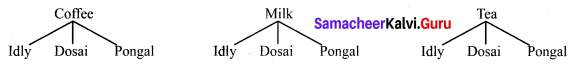So they have 9 combinations such as

1. Coffee and Idly
2. Coffee and Dosai
3. Coffee and Pongal
4. Milk and Idly
5. Milk and Dosai
6. Milk and Pongal
7. Tea and Idly
8. Tea and Dosai
9. Tea and Pongal.

Question 2.
Place the numbers from 1 to 12 exactly once in the circles so that the sum of numbers in each of the 5 lines of the star is 24.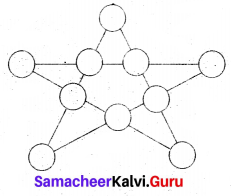Solution:
By trial and error, we arrange the numbers to get the sum 24.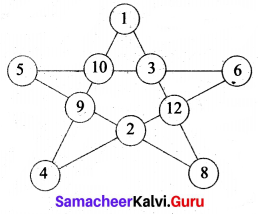Question 3.
Place the numbers from 1 to 12 in the circles without repetition to get a sum of 28, 29, 30 and 31.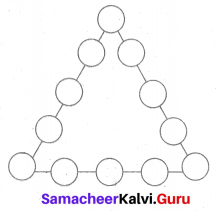Solution: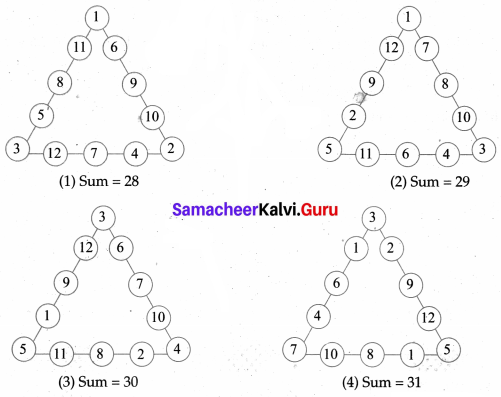Question 4.
Find the minimum number of straight lines used in forming the figure.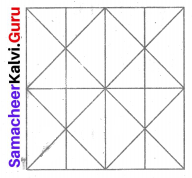Solution: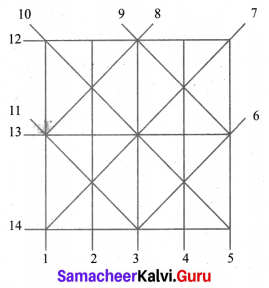The straight lines may be labelled as
Vertical lines = 5
Horizontal lines = 3
Standing lines = 6
Total of 14 lines are needed.

Question 5.
Find the dots in the 12th figure of the following: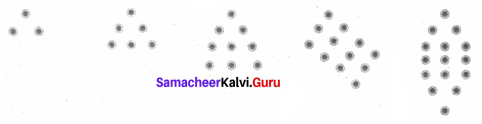Solution: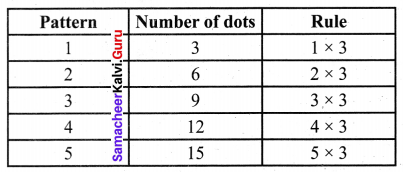10th figure will have 10 × 3 = 30 dots.
And 12th figure will have 12 × 3 = 36 dots.